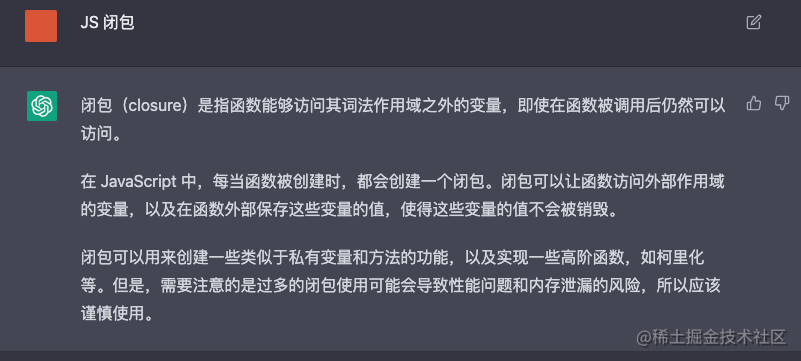# ChatGPT 来我司面试前端工程师，考考他闭包

## 第一问，说说 JS 闭包## 追问，详细讲讲闭包

1. 作用域

1. 词法作用域

1. 闭包的定义和作用

``````function outer() {let x = 1;function inner() {console.log(x);
}return inner;
}let closure = outer();closure(); // 1``````

1. 闭包的用途和注意事项

``````function counter() {let count = 0;return function() {
count++;console.log(count);
};
}let myCounter = counter();myCounter(); // 1myCounter(); // 2myCounter(); // 3``````

``````for (var i = 0; i < 10; i++) {setTimeout(function() {console.log(i); // 输出的都是 10
}, 1000);
}``````

## 那么如何解决在循环中使用闭包的陷阱

``````for (let i = 0; i < 10; i++) {setTimeout(function() {console.log(i); // 输出 0 到 9
}, 1000);
}``````

``````for (var i = 0; i < 10; i++) {
(function(j) {setTimeout(function() {console.log(j); // 输出 0 到 9
}, 1000);
})(i);
}``````

## let 的块级作用域讲讲

ES6 中引入了 `let` 关键字，它的一个重要特性就是可以创建块级作用域。这意味着，变量在块级作用域中声明，只能在该作用域内部访问，离开作用域后就会被销毁。

``````function myFunction() {if (true) {let x = 10;console.log(x); // 输出 10
}console.log(x); // 报错：ReferenceError: x is not defined
}``````

``````function myFunction() {if (true) {var x = 10;console.log(x); // 输出 10
}console.log(x); // 输出 10
}``````